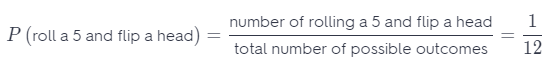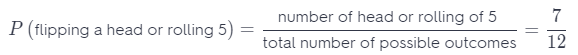# Texas Go Math Grade 7 Lesson 6.3 Answer Key Making Predictions with Theoretical Probability

Refer to our Texas Go Math Grade 7 Answer Key Pdf to score good marks in the exams. Test yourself by practicing the problems from Texas Go Math Grade 7 Lesson 6.3 Answer Key Making Predictions with Theoretical Probability.

## Texas Go Math Grade 7 Lesson 6.3 Answer Key Making Predictions with Theoretical Probability

Question 1.
Predict how many times you will roll a number less than 5 if you roll a standard number cube 250 times.
The probability of rolling number less than 5 is $$\frac{4}{6}$$ = $$\frac{2}{3}$$
Use proportion.
The probability of rolling number less than 5 is equal to what number “x divided by 150?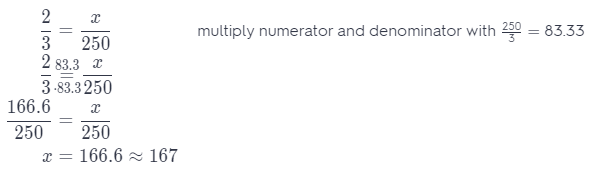To roll a number less than 5, you should roll a cube 167 times.

Texas Go Math Grade 7 Solutions Lesson 6.3 Answer Key Question 2.
You flip a fair coin 18 times. About how many times would you expect heads to appear?
The probability of flipping a coin and getting a head is $$\frac{1}{2}$$.
Use proportion.
The probability to flip a coin and get a head is equal to what number ‘x’ divided by 18?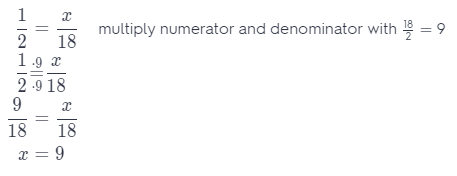To get a head, you should flip a coin 9 times.

Question 3.
A bag of marbles contains 8 red marbles, 4 blue marbles, and 5 white marbles. Tom picks a marble at random, Is it more likely that he picks a red marble or a marble of another color?
P (picking red marble) = $$\frac{8}{17}$$
P (picking a marble with another color) = 1 – P (picking red marble) = 1 – $$\frac{8}{17}$$ = $$\frac{9}{17}$$
The probability to pick red marble is less than a probability to pick a marbLe of another color because $$\frac{8}{17}$$ < $$\frac{9}{17}$$.

Question 4.
At a fundraiser, a school group charges $6 for tickets for a “grab bag.” You choose one bill at random from a bag that contains 40$1 bills, 20 $5 bills, 5$10 bills, 5 $20 bills, and 1$100 bill. Is it likely that you will win enough to pay for your ticket? Justify your answer.
Total number of possible outcomes is: 40 + 20 + 5 + 5 + 1 = 71.
Number of $10,$20 and $100 bills is 5 + 5 + 1 = 11.\$
P (win enough to pay ticket) =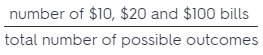= $$\frac{11}{71}$$
1 – P (win enough to pay ticket) = 1 –= 1 – $$\frac{11}{71}$$ = $$\frac{60}{71}$$
It is more likely not to win enough to pay a ticket.

Question 1.
Bob works at a construction company. He has an equally likely chance of being assigned to work different crews every day. He can be assigned to work on crews building apartments, condominiums, or houses. If he works 18 days a month, about how many times should he expect to be assigned to the house crew? (Example 1)
STEP 1 Find the probabilities of beg assigned to each crew.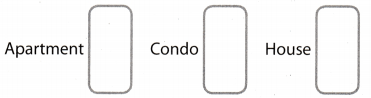The probability of being assigned to the house crew is _________.

STEP 2: Set up and solve a proportion.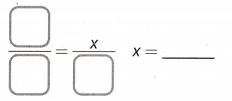Bob can expect to be assigned to the house crew about _______ times out of 18.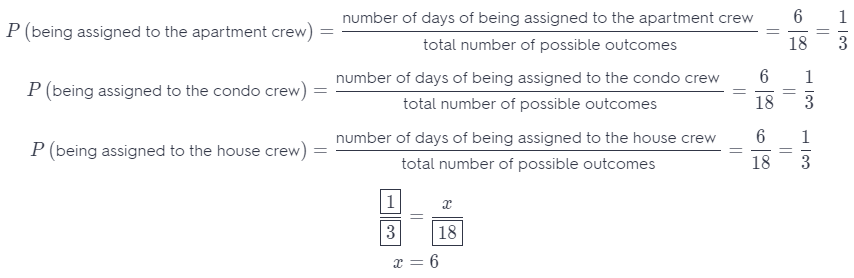The probability of being assigned to the house crew is $$\frac{1}{3}$$
Bob can expect to be assigned to the house crew about 6 times out of 18.

Making Predictions with Theoretical Probability Lesson 6.3 Answer Key Question 2.
During a raffle drawing, half of the ticket holders will receive a prize. The winners are equally likely to win one of three prizes: a book, a gift certificate to a restaurant, or a movie ticket. If there are 300 ticket holders, predict the number of people who will win a movie ticket. (Example 1)
P(win) = $$\frac{1}{2}$$
P (win a movie ticket) =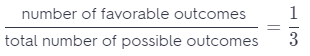Use a proportion.
$$\frac{1}{3}$$ = $$\frac{x}{150}$$
x = 50
About 50 peoples will win a movie tickets.

Question 3.
In Mr. Jawarani’s first period math class, there are 9 students with hazel eyes, 10 students with brown eyes, 7 students with blue eyes, and 2 students with green eyes. Mr. Jawarani picks a student at random. Which color eyes is the student most likely to have? Explain. (Example 2)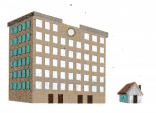Total number of possible outcomes is: 9 + 10 + 7 + 2 = 28.It is more likely to pick a student with brown eyes.

Essential Question Check-In

Question 4.
How do you make predictions using theoretical probability?
Because theoretical and experimental probabilities are ratios, we can use proportions with probabilities to make predictions. Therefore using an equation where the theoretical probability is equal to number of x divided by a total number of outcomes, we get a prediction for some event, which is number x.

Question 5.
A bag contains 6 red marbles, 2 white marbles, and 1 gray marble. You randomly pick out a marble, record its color, and put it back in the bag. You repeat this process 45 times. How many white or gray marbles do you expect to get?
Total. number of possibIe outcomes is: 6 + 2 + 1 = 9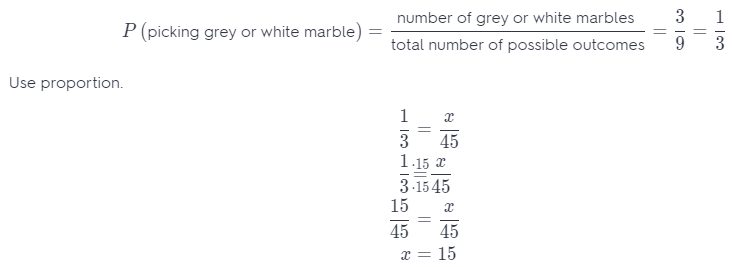You can expect about 15 grey or white marbles.

Using the blank circle below, draw a spinner with 8 equal sections and 3 colors—red, green, and yellow. The spinner should be such that you are equally likely to land on green or yellow, but more likely to land on red than either on green or yellow.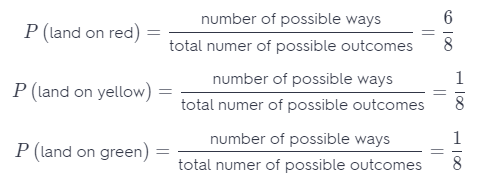P (land on red) = $$\frac{6}{8}$$
P (land on yellow) = $$\frac{1}{8}$$
P (land on green) = $$\frac{1}{8}$$

Use the following for Exercises 7-9.

In a standard 52-card deck, half of the cards are red and half are black. The 52 cards are divided evenly into 4 suits: spades, hearts, diamonds, and clubs. Each suit has three face cards (jack, queen, king), and an ace. Each suit also has 9 cards numbered from 2 to 10.

Question 7.
Dawn draws 1 card, replaces it, and draws another card. Is it more likely that she draws 2 red cards or 2 face cards?
Total number of possible outcomes is:
red-black, red-red, black-black, black-red = 4
P (draw two red cards) =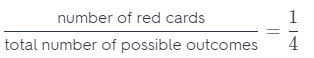Total number of possible outcomes is:
52 possibilities ∙ 52 possibilities = 52 ∙ 52 = 2704
P (draw two face cards) =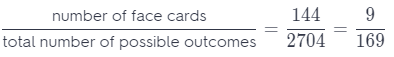The probability to pick two red cards is more likely than the probability to pick two face cards.

Question 8.
Luis draws 1 card from a deck, 39 times. Predict how many times he draws an ace.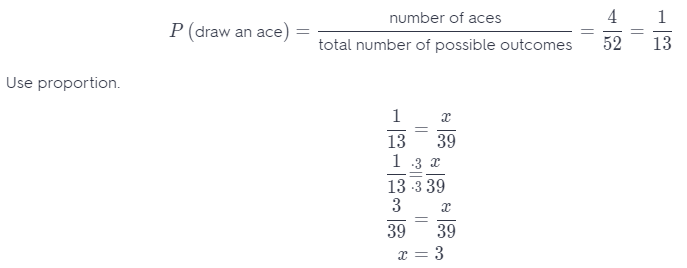You can predict that an ace will be drawn about 3 times.

Suppose a solitaire player has played 1,000 games. Predict how many times the player turned over a red card as the first card.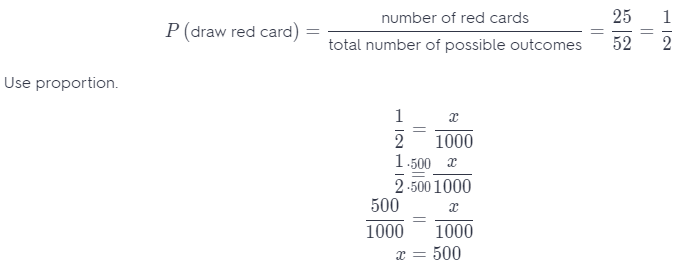You can predict that the player will, turn over the red card as the first card about 500 times.

Question 10.
John and O’Neal are playing a board game in which they roll two number cubes. John needs to get a sum of 8 on the number cubes to win. O’Neal needs a sum of 11. If they take turns rolling the number cube, who is more likely to win? Explain.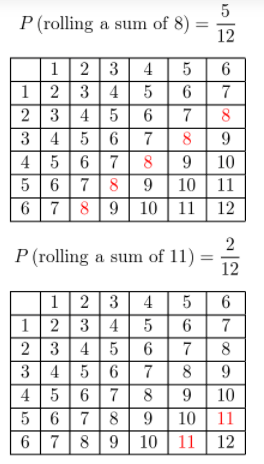It is more likely John to win.

Question 11.
Every day, Navya’s teacher randomly picks a number from 1 to 20 to be the number of the day. The number of the day can be repeated. There are 180 days in the school year. Predict how many days the number of the day will be greater than 15.You can expect the number of day will be greater than 5 about 45 days.

Go Math 7th Grade Lesson 6.3 Theoretical Probability Answer Key Question 12.
Eben rolls two standard number cubes 36 times. Predict how many times he will roll a sum of 4.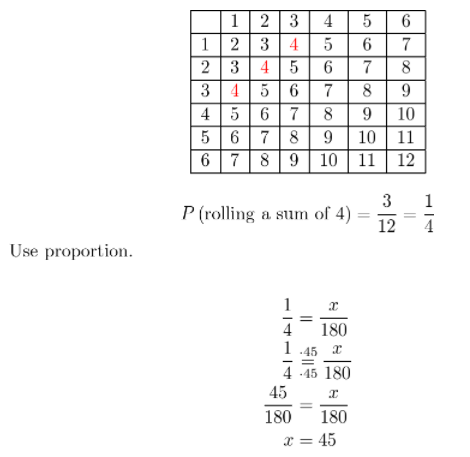About 45 times will roll a sum of 4.

Question 13.
Communicate Mathematical Ideas Can you always show that a prediction based on theoretical probability is true by performing the event often enough? If so, explain why. If not, describe a situation that justifies your response.
Example:
A coin is flipped 16 times and head is obtained 7 times. Find a experimental. probability to flip a head.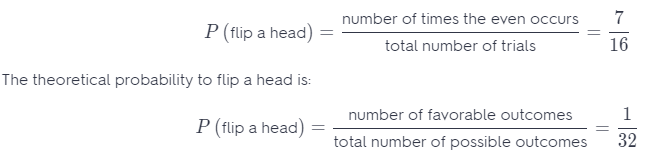Question 14.
Represent Real-World Problems Give a real-world example of an experiment in which all of the outcomes are not equally likely. Can you make a prediction for this experiment, using theoretical probability?
What is the probability of rolling a 2 or 5 on a 6-sided cube with one of next numbers on each side: 1, 2, 2, 3, 4,5?
How 2 appear on two sides of the cube, and 5 only on one, it is more likely that we will roll a 2.
Using the theoretical probability we will show the same.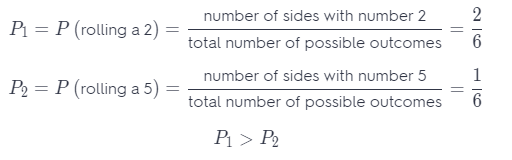H.O.T. Focus on Higher Order Thinking

Question 15.
Critical Thinking Pierre asks Sherry a question involving the theoretical probability of a compound event in which you flip a coin and draw a marble from a bag of marbles. The bag of marbles contains 3 white marbles, 8 green marbles, and 9 black marbles. Sherry’s answer, which is correct, is $$\frac{12}{40}$$. What was Pierre’s question?
Pierre’s question was: What is the probability to pick white or black marble and flip a head?

Go Math Grade 7 Lesson 6.3 Making Predictions Question 16.
Make a Prediction Horace is going to roll a standard number cube and flip a coin. He wonders if it is more likely that he rolls a 5 and the coin lands on heads, or that he rolls a 5 or the coin lands on heads. Which event do you think is more likely to happen? Find the probability of both events to justify or reject your initial prediction.
Total number of possible outcomes for rolling 5 and flip a head is: 6 possibilities ∙ 2 possibilities 6 ∙ 2 = 12
Number of rolling a 5 and flip a head is only one.